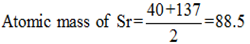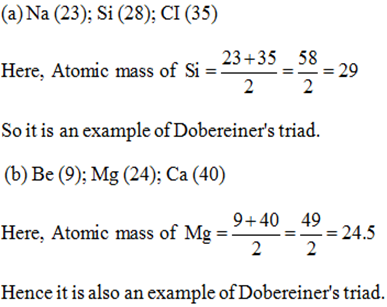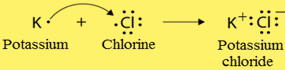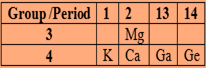# NCERT Exemplar Problems and their Solutions

Reviewed By:
Krishna Kant Majee
M.Sc., B.Ed.

### Chapter 5-Periodic Classification of Elements-Short Answers

Q.27. The three elements A, B and C with similar properties have atomic masses X, Y and Z respectively. The mass of Y is approximately equal to the average mass of X and Z. What is such an arrangement of element called as? Give one example of such a set of elements.
This is an example of Dobereiner’s triads which is a group of three elements. In Dobereiner’s triads the atomic masses of elements are arranged in such a way that the atomic mass of middle element is almost the mean of atomic masses of first and third elements.
For example; Ca, Sr and Ba is one of the Dobereiner’s triads. Here, atomic masses of Ca, Sr and Ba are 40, 88 and 137 respectively. Atomic mass of Sr that is 88 is almost the mean of atomic masses of Ca and Ba.Q.28. Elements have been arranged in the following sequence on the basis of their increasing atomic masses.
F, Na, Mg, Al, Si, P, S, Cl, Ar, K
(a) Pick two sets of elements which have similar properties.
(b) The given sequence represents which law of classification of elements?
(a) In this sequence, the elements are arranged in increasing order of their atomic masses. According to Newlands’ law of octaves, every eighth element will show similar properties as that of the first element. Hence F and Cl, Na and K will show similar properties.
Therefore, Set I is F and Cl
And, Set II is Na and K
(b) In the given sequence, elements are arranged in increasing order of their atomic masses and each 8th element shows similar properties. So, it represents the Newlands’ law of octaves.
F Na Mg Al Si P S CI Ar K
Q.29. Can the following groups of elements be classified as Dobereiner’s triad?
(a) Na, Si, Cl
(b) Be, Mg, Ca
Atomic mass of Be 9; Na 23; Mg 24; Si 28; Cl 35; Ca 40
Explain by giving reason.
For a group to be Dobereiner’s triad, the atomic mass of middle element must be average of atomic masses of the first and the third elements.Q.30 In Mendeleev’s periodic table, the elements were arranged in the increasing order of their atomic masses. However, cobalt with atomic mass of 58.93 amu was placed before nickel having an atomic mass of 58.71 amu. Give reason for the same.
In Mendeleev’s periodic table, the reasons for placing cobalt (Co) before nickel (Ni) though its atomic mass is higher than that of nickel, are:
(i) Cobalt was placed in the group of rhodium (Rh) and iridium (Ir) because it exhibits the properties similar to them.
(ii) Similarly Nickel was placed with elements like palladium (Pd) and platinum (Pt) having properties similar to it.
Q.31. ‘Hydrogen occupies a unique position in modern periodic table’, justify the statement.
The position of hydrogen is unique in Modern Periodic table. This is because:
(i) The valence shell configuration of hydrogen and alkali metals is same, contain one electron. That is the reason, some of the properties of hydrogen are similar to those of alkali metals and it must be placed group 1.
(ii) Both hydrogen and halogens require one electron to complete their valence configuration therefore they show similarities in their properties and hydrogen can be placed in group 17.
(iii)Hydrogen also exhibits some unique properties like oxides of hydrogen (water ) is neutral in nature whereas meals form basic oxides and halogens form acidic oxides.
Q.32. Write the formulae of chlorides of Eka-silicon and Eka-aluminium, the elements predicted by Mendeleev.
Eka-silicon is identified as germanium (Ge) which is placed in group 4 of Mendeleev’s periodic table. The valency of germanium is 4 so the chemical formula for chloride must be $GeCl_{4}$.
Eka-aluminium was later identified as gallium (Ga). It is placed in group 3 of the Mendeleev’s periodic table. Hence the valence of gallium is 3 and the formula of chloride would be $GaCl_{3}$.
Q.33 Three elements A, B and C have 3, 4 and 2 electrons respectively in their outermost shell. Give the group number to which they belong in the modern periodic table. Also, give their valencies.
The group number for elements can be identified as 10 + number of valence electrons. Element A with 3 valence electrons must show valency as 3 and should belongs to group 13. The elements of group 13 are B, Al, Ga, In or Tl.
Element B contains 4 valence electrons so it must be from group 14 ( 10 +4) and valency should be 4. The element B can be C, Si, Ge, Sn or Pb. Similarly Element C has two valence electrons hence must be from group 2. So element C can be Be, Mg, Ca, Sr, Ba or Ra.
Q.34. If an element X is placed in group 14, what will be the formula and the nature of bonding of its chloride?
Elements of group 14 have 4 valence electrons and valency would be 4. Group 14 contains metals and non-metals with metalloids.
As there are four valence electrons so it must involve in covalent bonding to complete its octet configuration. So the chemical formula of chloride of element X would be$XCI_{4}$.
Since chloride is formed by sharing of electrons therefore the nature of the chloride of element X will be covalent.
(a) X has 12 protons and 12 electrons
(b) Y has 12 protons and 10 electrons
Here X has same number of electrons and protons, therefore it is a neutral atom whereas Y contains 12 protons and 10 electrons so there are 2 protons extra giving Y a charge of +2. The electronic configurations of the two species can be expressed as:

 X Y KL    M KL 28     2 28

The atomic size of a cation is always smaller than a neutral atom containing same number of protons this is because cation has less number of electrons due to which the nuclear attractions are more on electrons in cation causing its size to be smaller than that of the neutral atom.
Hence, atomic radius of Y is smaller than that of X.
Q.36. Arrange the following elements in increasing order of their atomic radii
(a) Li, Be, F, N
(b) Cl, At, Br, I
(a) Li, Be, F and N belong to same period of modern periodic table. As we move left to right in the periodic table, the atomic radii of elements decreases due to high atomic charge and same number of shell. Thus the atomic radii of Li, Be, F and N increase in the order: F < N < Be < Li.
(b) Cl, At, Br and I belong to same group of periodic table that is group 17. As we move down in a group, the atomic radii of elements increase due to increasing the number of shell. Thus, atomic radii of Cl, At, Br and I increase in the order: Cl < Br < I < At.
Q.37. Identify and name the metals out of the following elements whose electronic configurations are given below.
(a) 2, 8, 2
(b) 2, 8, 1
(c) 2, 8, 7
(d) 2, 1
Elements with having 1 to 3 valence electrons are usually metals whereas elements with 4 or more valence electrons are usually non-metals or metalloids.
Therefore, from given electronic configurations, metals are:
(a) 2, 8, 2 – Magnesium (12)
(b) 2, 8, 1 – Sodium (11)
(d) 2, 1 – Lithium (3)
Q.38. Write the formula of the product formed when the element A (atomic number 19) combines with the element B (atomic number 17). Draw its electronic dot structure. What is the nature of the band formed?
The electronic configuration of element A with atomic number 19 would be 2, 8, 8, 1. Hence it is potassium. Since, it has omly one valence electron therefore it must be a metal.
The electronic configuration of element B would be 2, 8, 7 with number of valence electrons being7. So, it must be a non-metal that is chlorine.
A metal and a non-metal usually combine through ionic bond because metals have tendency to lose electrons and form cations whereas non-metals can accept electrons to form anions.
Potassium and chlorine will form potassium chloride (KCl).
The electron dot structure of KCl is as given belowQ.39. Arrange the following elements in the increasing order of their metallic character Mg, Ca, K, Ge, Ga.
Answer. The arrangement of given elements in the Modern Periodic table is listed below.We know that metallic character decreases from left to right in the periodic table. In the given elements, excluding Mg, remaining elements are placed in same period so the metallic character will decrease from K to Ge and order of metallic character would be;
Ge < Ga < Ca < K
Now, as we move down in a group, metallic nature of elements increases. So, Ca is more metallic than Mg. Similarly, Mg will be less metallic than K because in period, metallic nature decreases from left to right. Hence the increasing order of metallic character would be;
Ge < Ga < Mg < Ca < K
Q.40. Identify, the elements with the following property and arrange them in increasing order of their reactivity.
(a) An element which is a soft and reactive metal.
(b) The metal which is an important constituent of limestone.
(c) The metal which exists in liquid state at room temperature.
(a) Alkali metals like sodium and potassium are soft and reactive.
(b) The chemical formula of limestone is $CaCO_{3}$ hence the important constituent of limestone would be calcium (Ca).
(c) Mercury is the only metal which exists in liquid state at room temperature.
Since reactivity of metals decreases from left to right in the group therefore the order of reactivity of Na, Ca and Hg would be;
Hg < Ca < Na
Q.41. Properties of the elements are given below. Where would you locate following elements in the periodic table?
(a) A soft metal stored under kerosene.
(b) An element with variable (more than one) valency stored under water.
(c) An element which is tetravalent and forms the basis of organic chemistry.
(d) An element which is an inert gas with atomic number 2.
(e) An element whose thin oxide layer is used to make other elements corrosion resistant by the process of “anodising”.
(e) Aluminium metal is used to make other elements corrosion resistant by the process of “anodising”. The oxide layer is of $Al_{2}O_{3}$. The atomic number of aluminum is 13 and it is placed in group 13 and period 3 of periodic table.